# Numerical Study of the Surface Roughness, Thermal Conductivity of the Contact Materials and Interstitial Fluid Convection Coefficient Effect on the Thermal Contact Conductance

Numerical Study of the Surface Roughness, Thermal Conductivity of the Contact Materials and Interstitial Fluid Convection Coefficient Effect on the Thermal Contact Conductance

Chadouli RachidFrédéric Lebon Iulian Rosu Makhlouf Mohammed

Technology Department, Faculty of Science and Technology, Djilili Bounaama University, Khemis Miliana 44225, Algeria

Aix Marseille University, Centre National de la recherche Scientifique, Centrale Marseille, Laboratoire de Mécanique et d’acoustique, Marseille 13453, France

Mechanical Engineering Department, Faculty of Technology, Djilili Liabes University, Sidi Bel Abbes 22000, Algeria

Corresponding Author Email:
Page:
265-271
|
DOI:
https://doi.org/10.18280/acsm.430410
20 May 2019
|
Accepted:
2 August 2019
|
Published:
28 September 2019
| Citation

OPEN ACCESS

Abstract:

In this paper, a numerical simulation by the computer code ABAQUS (6.14) was conducted to study the influence of the thermal conductivity, the interstitial fluid convection coefficient and surface roughness on the thermal contact conductance "TCC" to improve the heat transfer between the materials in contact. In our study, the pairs specimens involved have a thermal conductivity k ranging between 20 w/m·K to 390 w/m·K. The diameter of specimen d is 2 cm, the height L is 8 cm, and the surface roughness Ra is in the range of 5 to 20µm. Results indicated that the surface roughness and thermal conductivity present a power law relationship with heat transfer enhancement between solids in contact. Moreover, the interstitial fluid convection coefficient influences weakly the variation in the thermal contact conductance. The TCC magnitude increase more rapidly with decrease of the specimen’s surface roughness when the latters have high thermal conductivity than when they have low thermal conductivity.

Keywords:

thermal contact conductance, thermal conductivity, convection coefficient, roughness, interstitial fluid

1. Introduction

The thermal contact resistance which is defined as the inverse of the thermal contact conductance “TCC” is the resistance exerted to the heat flow between two materials in contact due to the irregular geometry of surfaces, this irregularity makes contact occurs only at a few points that are called asperities, separated from each other by an interstitial space. The contact taking place only through surface asperities, so the lines of thermal flux at the contact region are moving towards these points "converging flux lines", this phenomena is known as constriction,other hand, the interstitial medium is generally , will affect a and reduce heat transfer, temperature at contact.

A large number of theoretical and experimental studies have been performed on contact heat transfer since 1980s.

The objective of the present study is dedicated to compute the thermal contact conductance (TCC) between rough surfaces and to describe the impact of the size and shape of the surface roughness, thermal conductivity of the materials and the interstitial fluid convection coefficient on the TCC. The commercial software Abaqus (6.14) was used to compute numerically the TCC between rough surfaces as function of the contact specimen’s thermal conductivity, interstitial fluid convection coefficient, size and shape of the roughness surfaces using the Finite Element Method. It should be noted that the previous works in this field studies the effect of the interstitial medium and seal without taking into account the quality of the contact materials and the contact interface geometry .

In the literature, most of numerical and experimental studies based on the area contact thickness effect [13-14], contrarily, there has not been any work on the interstitial medium thermal conductivity effect which is the subject of our study.

The paper is decomposed as follow: Section 2 is devoted to the methodology. Firstly, a statement of the problem is presented. Secondly, a numerical procedure based on the Finite Element Method is proposed. In section 3, numerical results are presented: first, the effect of the roughness surface, thermal conductivity and interstitial fluid convection coefficient on the TCC are studied, then the actual contact ratio and geometric shape of asperities effects are analyzed. The paper ends with conclusions and perspective.

2. Methodology

2.1 Statement of the problem

From Fourier law for steady state heat transfer by conduction, the thermal contact conductance TCC can be defined [6-10-11] by:

$T C C=\frac{Q}{T_{c h}-T_{f}}$ (1)

With $Q=\frac{1}{2}({{Q}_{ch}}+{{Q}_{f}})=\frac{1}{2}(-k\frac{d{{T}_{ch}}}{dz}-k\frac{d{{T}_{f}}}{dz})$  (2)

where, Q is the average flow of heat between the hot (Qch) and cold (Qf) specimens (W/m2); Tch and Tf are the temperatures of hot and cold specimens near the contact interface (see Figure 2), which are measured numerically.

The assumption of the average heat flux is present because we cannot compute the heat flux at the contact region, which contains two different media that are the asperities in contact and the interstitial fluid, this region, will undergo a temperature jump shown in the Figure 1.

## 1.png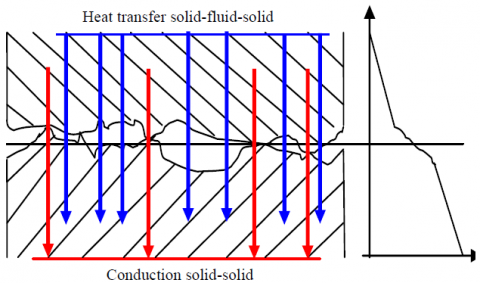Figure 1. Heat transfer between two solids with imperfect contact

2.2 Numerical model

The contact between two rough surfaces is modeled by the contact between rough surfaces with square form asperities.

In a first study and in order to investigate the effect of the roughness surface, thermal conductivity of contact materials and interstitial convection coefficient, a 2D steady state model has been performed using the Finite Element Method and obtained by Abaqus (6.14). The width of the two contacting bodies is 2 cm and the height is 8cm.The computation is based on the mesh convergence technique. Free and independent mesh types have been considered with standard elements and linear geometry, the mesh element are quadrilateral dominated form (DC2D4). The heat Transfer propagates vertically along the length of the two cylinder so the Z axis (see Figure 2) and not horizontally depending on the width of the cylinder, that is why the symmetry is not taken into account. All meshes are particularity refined close to the contact interfaces, but are sufficiently large away from the contact region. In this part, the same roughness and thermal conductivity have been considered and the asperities are square shaped with size ranging from 5 to 20 µm. The actual contact area is about 6-8 % of the nominal contact area for polished surfaces . The number of elements is n= 29752×2= 59504 elements.

In order to compute the thermal contact conductance, the upper face of the specimen 1 is heated at 538 K and the lower face of the specimen 2 is heated at 338 K. The two materials in contact are maintained initially at 298 K and the lateral surfaces are thermally insulated, so the heat transfer occurs only in the Z direction from top to bottom across the contact area.

From Eq. (1) and Eq. (2), both Tch and Tf temperatures can be evaluated numerically in order to compute the heat flow and the thermal contact conductance.

In the second part of study, the same model is study to investigate the geometric shape of asperities effect and contact rate effect on the thermal contact conductance.  For this purpose, a contact model with square, triangular, spherical and elliptical asperities shape it was used.

## 2.pngFigure 2. Geometrical model of roughness contact

3. Result and Discussion

3.1 Effect of the surface roughness Ra

In order to describe the effect of the surface roughness Ra on the thermal contact conductance TCC, a first case of a solid-solid rough contact with square asperities is studied. Four pairs of specimens were used in this study. Each pairs has the same surface roughness which ranges from 5 µm to 20 µm and same thermal conductivity which ranges from 20 to 390 W/m·K.

## 3.png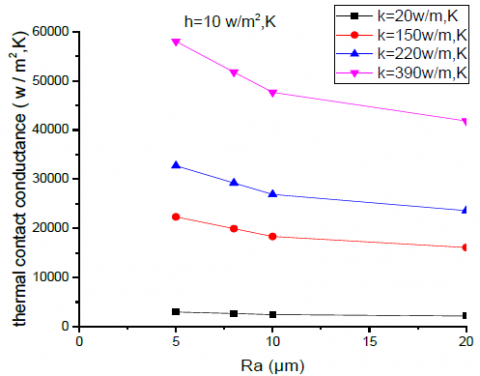Figure 3. Effect of the surface roughness on the TCC for different contact materials

Figure 3 shows the variation of the thermal contact conductance as a function of the surface roughness for the four pairs of specimens. The obtained results indicate that the thermal contact conductance is strongly influenced by the surface roughness for the materials which have a high thermal conductivity (k = 390 W/m2·K) unlike those that have a low conductivity (k = 20 W/m2·K) which varies slightly (Figure 3).

Figure 4 shows that the interstitial fluid convection coefficient has a low effect on the thermal contact conductance. Therefore, the TCC presents a power lower relationship with the surface roughness and the change of TCC is significant for high thermal conductivity specimens.

## 4.jpg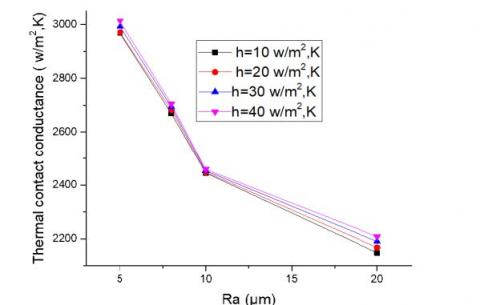Figure 4. Effect of the surface roughness on the TCC for different interstitial fluid convection coefficient

3.2 Effect of the thermal conductivity

In order to determine the relationship of the thermal conductivity k on the thermal contact conductance TCC, the study is performed with 4 pairs of specimens with different surface roughness and different interstitial fluid convection coefficients. The results are shown in Figure 5. It is concluded that the thermal contact conductance is strongly related to the thermal conductivity, especially for low thermal conductivity contact materials.

3.3 Effect of the interstitial fluid convection coefficient

In order to investigate the effect of the convection coefficient on the TCC, growth rates of the thermal contact conductance are compared as a function of the interstitial fluid convection coefficient for two different rough contacts.

The results show low relative change for polished surfaces (Ra =5 µm) and it is estimated at 1.48 % compared to the rough surfaces (Ra = 20 µm) which is estimated at 2.75 %. This difference is due to the considerable volume of interstitial fluid in the case of the rough contact with Ra = 20 µm.

Figure 6a and Figure 6b show the evolution of the heat transfer coefficient as a function of the interstitial fluid convection coefficient. It is noted that the TCC increases with the increasing of the interstitial fluid convection coefficient.

From Figure 7a, Figure 7b its concluded that for all surface roughness and contact materials, the convection coefficient of the interstitial fluid “h” affects slightly on the TCC.

3.4 Effect of the actual CONTACT ratio S*

The actual contact surface rate is defined as the ratio between the actual contact area Ar represented by the surface of the contact points and apparent contact area Aa represented by the width of the specimens used in this study (2 cm).

$S^{*}=\frac{A_{r}}{A_{a}}$   (3)

The evolution of the real contact area between two solids can be defined by flattening of the asperities peaks due to the application of a load which is called contact pressure, the objective of this study is to know how varies the thermal contact conductance as a function of improving the contact surface and not in function of the contact pressure. The study was conducted between two rough solids made of stainless steel with four geometric shape asperities: square, triangular, spherical and elliptical considering that interstitial fluid is air at 298 K with h = 10 W/m2·K.

## 5.png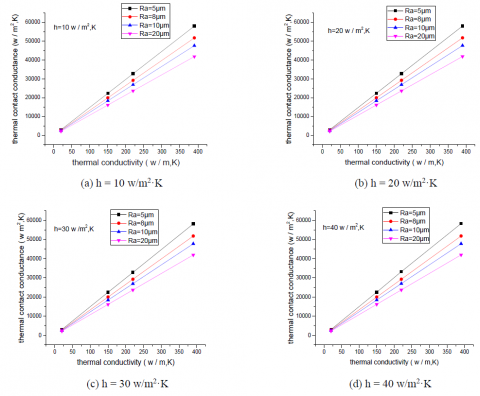Figure 5. Effect of the thermal conductivity on the TCC for different interstitial fluid convection coefficient

## 6.png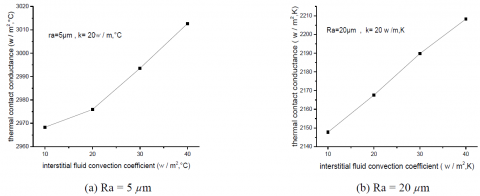Figure 6. Effect of the interstitial fluid convection coefficient on the TCC for different surfaces roughness

## 7.png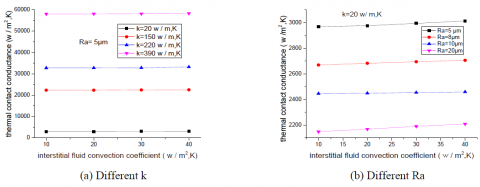Figure 7. Comparison of the interstitial fluid convection coefficient effect on the TCC for different k and Ra

## 8.pngFigure 8. Effect of the contact actual ratio S* on the TCC for different shape asperities

Figure 8a, 8b, 8c and 8d show that the TCC increases with increasing S*, so the heat transfer enhancement is strongly influenced by the rate of actual contact compared to convection coefficient interstitial fluid which affect slightly (Figure 9). Figure 9 shows the little impact of convection coefficient on the TCC except for the contact S*=10%, which significantly affects compared to other contact rate, this relative increase is of about 3 % in the case of S * = 10 % and does not exceed 0.4 % for the other contact. This difference can be justified by the considerable volume of interstitial fluid in the case of S*=10 %.

It can be concluded that the interstitial fluid convection coefficient has a low impact on the heat transfer coefficient for all contact rates.

By comparing the growth rate in thermal contact conductance as a function of the rate contact for the four forms of asperities, it is noted that the contact with square shape asperities provides a significant evolution of about 13.53 %, by against; the contact with elliptical forms asperities gives a low relative change of about 2.34 %. This difference can be justified by the large volume of the gap present in the case of contact with square shape asperities which decreases significantly when increasing of the contact rate S*.

It is very remarkable that for the contact with elliptical asperities, the TCC reaches its maximum starting from 60% which can be justified by the maximum reduction of the interstitial volume (fig 8.c).

## 9.png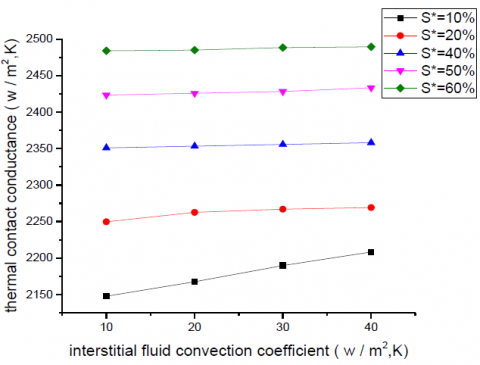Figure 9. Comparison of the actual contact ratio effect on the TCC

3.5 Effect of the geometric shape of asperities

In order to study the impact of the geometric shape of rough contact surfaces on the thermal contact conductance, the heat transfer coefficient was computed as function of the thermal conductivity of contact materials for four shapes: square, triangular, semi-spherical and semi-elliptical, considering that the interstitial fluid is air with h=10 w/m2.K at 298 K.

Figure 10 shows the geometric interface contact models used in the numerical computations of the thermal contact conductance (TCC).

It was observed that the thermal contact conductance has a relationship with the asperities shape. The TCC magnitude is significant for materials that have a high thermal conductivity (Figure 11).

The rough contact in the case of semi-elliptical asperities provides good thermal conduction at the contact, which can be justified by the low volume of the interstitial space between these asperities. The rough contact with square asperities contains a large interstitial volume compared to other types of asperities so it gives a little thermal contact conductance.

## 10.png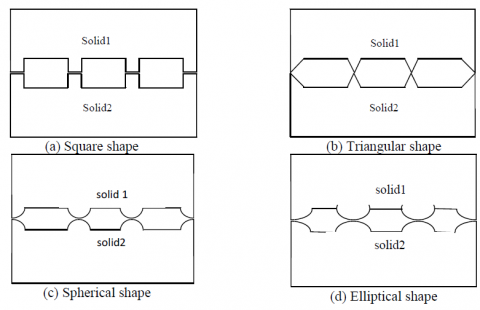Figure 10. Rough contact models used for the comparative study of the asperities shape effect on the TCR

## 11.png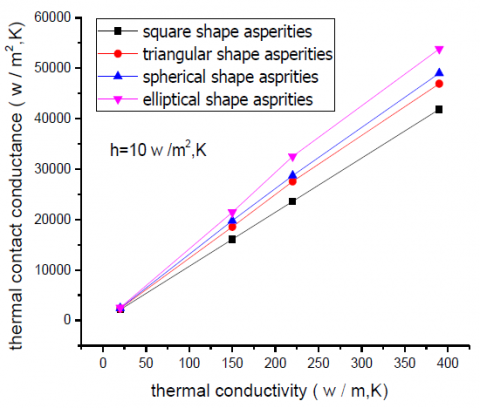Figure 11. Effect of the geometric shape of asperities on the TCC

4. Conclusions

In this study, and in order to the heat transfer between two rough surfaces, the thermal contact conductance at the interface as function of the size and shape of the surface roughness, thermal conductivity of the materials and the convective coefficient of the interstitial fluid was computed.

The results reveal that:

(1) The effect of surface roughness on the thermal contact conductance is highly noticed, which increases greatly in the case of polished surfaces.

(2) The thermal contact conductance increases with increasing of thermal conductivity, this increase is significant for large values of thermal conductivity.

(3) The impact of the interstitial fluid convection coefficient is very low on the thermal contact conductance especially for highly polished surfaces a small gap.

(4) Increasing the contact surface increases the heat transfer coefficient, which causes the decrease in the volume

(5) The shape of asperities has a strongly effect on the heat transfer coefficient especially for high thermal conductivity materials, the best values of this coefficient were obtained for a contact has semi-elliptic .

We recommend:

(1) To develop a theoretical model taking into account the roughness effects, thermal conductivity of solids in contact and convection coefficient of the interstitial fluid in order to introduce a function relating these parameters.

(2) To make an experimental study in order to extend the range of roughness and thermal conductivity.

Nomenclature
 A Surface, (m2) D Width, (m) k Thermal conductivity, (W/m·K) H Convection coefficient, W/m2·K Q Heat flux, (W/m2) S* Actual contact rate Ra Surface Roughness, (µm) T Temperature, (K) TCC Thermal contact conductance  (W/m2·K) ΔT Jump temperature, (K) z Coordinate, (m) Subscripts 1 Solid 1 2 Solid 2 A Area R reel Ch Hot F Cold
References

 Dou, R.F., Ge, T.R., Liu, X.L., Wen, Z. (2016). Effects of contact pressure, interface temperature, and surface roughness on the thermal contact conductance between stainless steel surface under atmosphere condition. International Journal of Heat and Mass Transfer, 94: 156-163. http://dx.doi.org/10.1016/j.ijheatmasstransfer.2015.11.069

 Sponagle, B., Groulx, D. (2016). Measurement of thermal interface conductance at variable clamping pressures using a steady state method. Applied Thermal Engineering, 96: 671-681. http://dxdoi.org/10.1016/j.appithermaleng.2015.12.010

 Tang, Q.Y., He, J.J., Zhang, W.F. (2015). Influencing factors of thermal contact conductance between TC4/30CrMnSi interfaces. International Journal of Heat and Mass Transfer, 86: 694-698. http://dx.doi.org/10.1016/j.ijheatmasstransfer.2015.03.035

 Cames-Pintaux, A.M., Padet, J.P. (1980). Study of thermal transient contacts. Proposal of a thermally equivalent model. International Journal of Heat and Mass Transfer, 23: 981-990.

 Verma, N.N., Mazumder, S. (2016). Extraction of thermal contact conductance of metal–metal contacts from scale-resolved direct numerical simulation. International Journal of Heat and Mass Transfer, 94: 164-173. http://dx.doi.org/10.1016/j.nucengdes.2015.11.026

 Zhu, Z., Zhang, L.W., Wu, Q.K., Gu, S.D. (2013). An experimental investigation of thermal contact conductance of Hastelloy C-276 based on steady state heat flux method. International. Communication in Heat and Mass Transfer, 41: 63-67. http://dx.doi.org/10.1016/j.icheatmasstransfer.2012.11.007

 Dureja, A.K., Pawaskar, D.N., Sesbou, P., Sinha, S.K., Sinha, R.K. (2015). Experimental determination of thermal contact conductance between pressure and calandria tubes of Indian pressured heavy water reactors. Nuclear Engineering and Design, 284: 60-66. http://dx.doi.org/10.1016/j.nucengdes.2014.11.025

 Wang, Z.R., Yang, J, Yang, M.Y., Zhang, W.F. (2012). Investigation on thermal contact conductance based on data analysis method of reliability. Chinese Journal of Aeronautics, 25(5): 791-795. http://dx.doi.org/10.1016/S1000-9361(11)60446-9

 Xu, R.P., Feng, H.D., Zhao, L.P., Xu, L. (2006). Experimental investigation of thermal contact conductance at low temperature based on fractal description. International Communications in Heat and Mass Transfer, 33(7): 811-818. https://doi.org/10.1016/j.icheatmasstransfer.2006.02.023

 Xu, R.P., Xu, L. (2005). An experimental investigation of thermal contact conductance of stainless steel at low temperatures. Cryogenics, 45(10-11): 694-704. http://dx.doi.org/10.1016/j.cryogenics.2005.09.002

 Gopal, V., Whiting, M.J., Chew, J.W., Mills, S. (2013). Thermal contact conductance and its dependence on load cycling. International Journal of Heat and Mass Transfer, 66: 444-450. http://dx.doi.org/10.1016/j.ijheatmasstransfer.2013.06.061

 Chadouli, R., Lebon, F., Rosu, I., Makhlouf, M. (2017). Numerical study of the gasket thermal conductivity effect on the thermal contact resistance between two solids in contact. Frontiers in Heat and Mass Transfer. http://dx.doi.org/10.5098/hmt.8.30

 Zhang, X.Z., Zhang, L.W., Xing, L. (2010). Study of thermal interfacial resistance between TC11/Glass Lubrication/K403 joint. Experimental Thermal and Fluid Science, 34(1): 48-52. http://dx.doi:10.1016/j.expthermflusci.2009.09.001

 Voller, G.P., Tirovic, M. (2007). Conductive heat transfer across a bolted automotive joint and the influence of interface conditioning. International Journal of Heat and Mass Transfer, 50(23-24): 4833- 4844. http://dx.doi.org/10.1016/j.ijheatmasstransfer.2007.03.001

 Madhusudana, C.V. (1996). Thermal contact conductance, Springer-Verlag. Berlin, Germany.